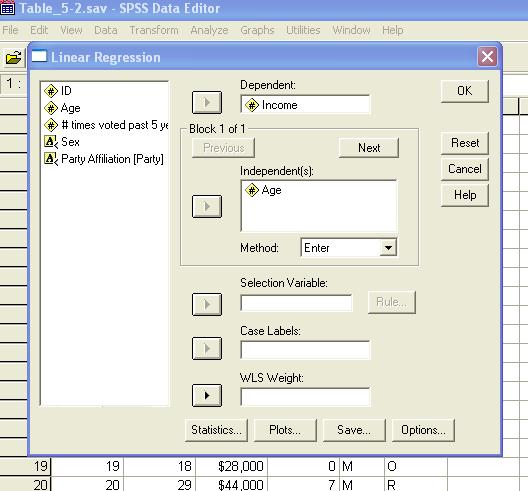﻿ Linear Regression using SPSS for Windows #1Graziano & Raulin
Research Methods (8th edition)

## Correlation and Regression

This screen shows the Regression box. To compute the regression line for predicting income from age, move the variable age to the independent variable box and the variable income to the dependent variable box and click on OK.

Note that it is possible to have more than one independent variable used in the prediction of the dependent variable. This is called multiple regression. To compute a multiple regression, you would enter as many independent variables as you would like. You should then choose a method. The two most commonly used methods are listed as "Enter" and "Stepwise."

The enter method puts all independent variables into the equation at once. The stepwise method puts the best independent variable in first, then does computations to determine which of the remaining variables will do the most to improve the prediction and that variable is entered. This process is continued until all of the independent variables have been entered. Multiple regression techniques are complex, and we recommend that you not use them unless you have read about or studied the options or have consulted with a statistical expert.